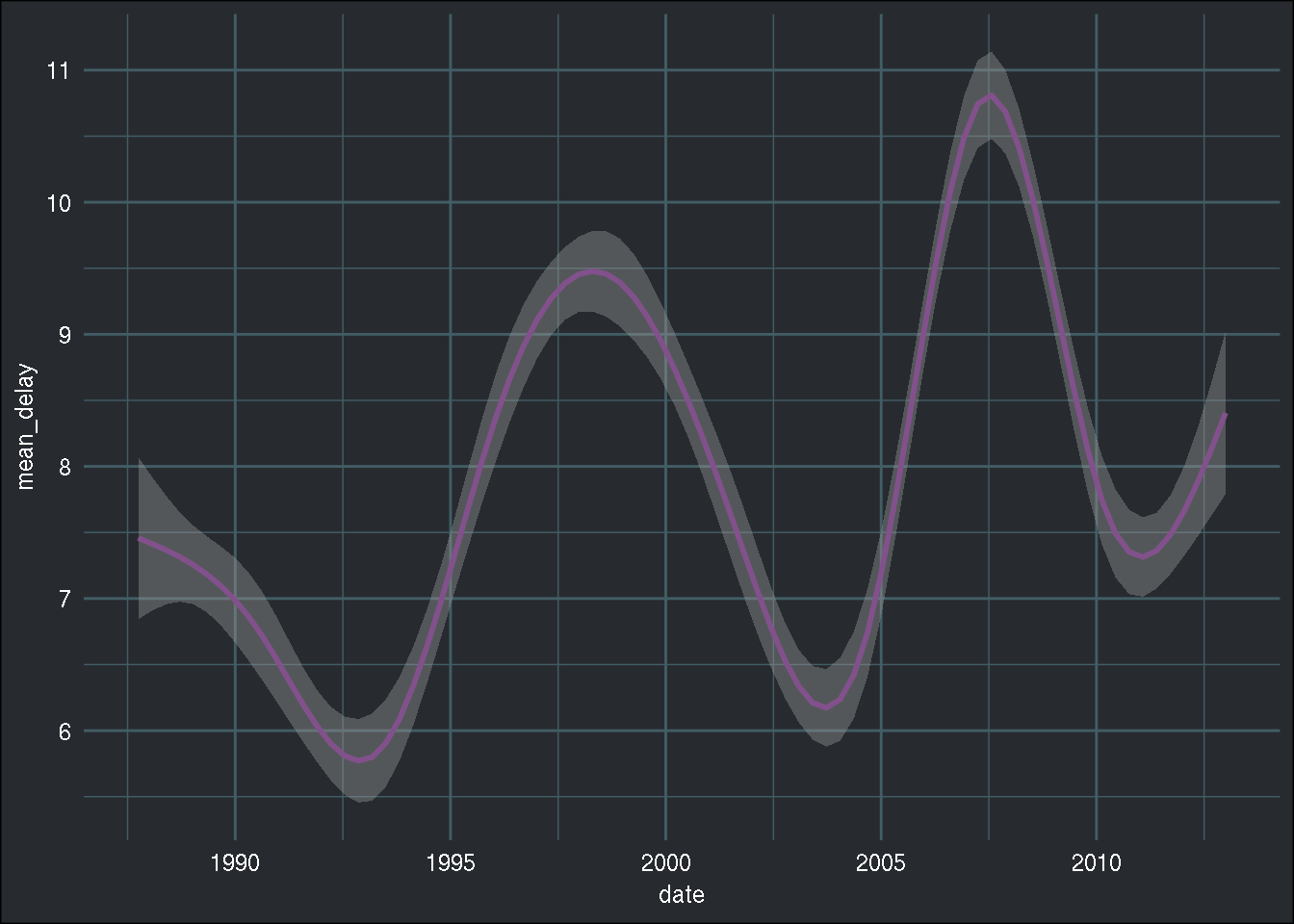Have a question about one of the blog posts? Chat here with me.

# {disk.frame} is epic

R

Note: When I started writing this blog post, I encountered a bug and filed a bug report that I encourage you to read. The responsiveness of the developer was exemplary. Not only did Zhuo solve the issue in record time, he provided ample code snippets to illustrate the solutions. Hats off to him!

This blog post is a short presentation of `{disk.frame}` a package that makes it easy to work with data that is too large to fit on RAM, but not large enough that it could be called big data. Think data that is around 30GB for instance, or more, but nothing at the level of TBs.

I have already written a blog post about this topic, using Spark and the R library `{sparklyr}`, where I showed how to set up `{sparklyr}` to import 30GB of data. I will import the same file here, and run a very simple descriptive analysis. If you need context about the file I’ll be using, just read the previous blog post.

The first step, as usual, is to load the needed packages:

``````library(tidyverse)
library(disk.frame)``````

The next step is to specify how many cores you want to dedicate to `{disk.frame}`; of course, the more cores you use, the faster the operations:

``````setup_disk.frame(workers = 6)
options(future.globals.maxSize = Inf)``````

`setup_disk.frame(workers = 6)` means that 6 cpu threads will be dedicated to importing and working on the data. The second line, `future.globals.maxSize = Inf` means that an unlimited amount of data will be passed from worker to worker, as described in the documentation.

Now comes the interesting bit. If you followed the previous blog post, you should have a 30GB csv file. This file was obtained by merging a lot of smaller sized csv files. In practice, you should keep the files separated, and NOT merge them. This makes things much easier. However, as I said before, I want to be in the situation, which already happened to me in the past, where I get a big-sized csv file and I am to provide an analysis on that data. So, let’s try to read in that big file, which I called `combined.csv`:

``````path_to_data <- "path/to/data/"

flights.df <- csv_to_disk.frame(
paste0(path_to_data, "combined.csv"),
outdir = paste0(path_to_data, "combined.df"),
in_chunk_size = 2e6,
backend = "LaF")``````

Let’s go through these lines, one at a time. In the first line, I simply define the path to the folder that contains the data. The next chunk is where I read in the data using the `csv_to_disk_frame()` function. The first option is simply the path to the csv file. The second option `outdir =` is where you need to define the directory that will hold the intermediary files, which are in the fst format. This folder, that contains these fst files, is the `disk.frame`. fst files are created by the `{fst}` package, which provides a fast, easy and flexible way to serialize data frames. This means that files that are in that format can be read and written much much faster than by other means (see a benchmark of `{fst}` here). The next time you want to import the data, you can use the `disk.frame()` function and point it to the `combined.df` folder. `in_chunk_size =` specifies how many lines are to be read in one swoop, and `backend =` is the underlying engine that reads in the data, in this case the `{LaF}` package. The default backend is `{data.table}` and there is also a `{readr}` backend. As written in the note at the beginning of the blog post, I encourage you to read the github issue to learn why I am using the `LaF` backend (the `{data.table}` and `{readr}` backend work as well).

Now, let’s try to replicate what I did in my previous blog post, namely, computing the average delay in departures per day. With `{disk.frame}`, one has to be very careful about something important however; all the `group_by()` operations are performed per chunk. This means that a second `group_by()` call might be needed. For more details, I encourage you to read the documentation.

The code below is what I want to perform; group by day, and compute the average daily flight delay:

``````mean_dep_delay <- flights.df %>%
group_by(YEAR, MONTH, DAY_OF_MONTH) %>%
summarise(mean_delay = mean(DEP_DELAY, na.rm = TRUE))``````

However, because with `{disk.frame}`, `group_by()` calls are performed per chunk, the code must now be changed. The first step is to compute the sum of delays within each chunk, and count the number of days within each chunk. This is the time consuming part:

``````tic <- Sys.time()
mean_dep_delay <- flights.df %>%
group_by(YEAR, MONTH, DAY_OF_MONTH) %>%
summarise(sum_delay = sum(DEP_DELAY, na.rm = TRUE), n = n()) %>%
collect()
(toc = Sys.time() - tic)
Time difference of 1.805515 mins``````

This is pretty impressive! It is much faster than with `{sparklyr}`. But we’re not done yet, we still need to compute the average:

``````mean_dep_delay <- mean_dep_delay %>%
group_by(YEAR, MONTH, DAY_OF_MONTH) %>%
summarise(mean_delay = sum(sum_delay)/sum(n))``````

It is important to keep in mind that `group_by()` works by chunks when dealing with `disk.frame` objects.

To conclude, we can plot the data:

``````library(lubridate)
dep_delay <- mean_dep_delay %>%
arrange(YEAR, MONTH, DAY_OF_MONTH) %>%
mutate(date = ymd(paste(YEAR, MONTH, DAY_OF_MONTH, sep = "-")))

ggplot(dep_delay, aes(date, mean_delay)) +
geom_smooth(colour = "#82518c") +
brotools::theme_blog()``````
``## `geom_smooth()` using method = 'gam' and formula 'y ~ s(x, bs = "cs")'```{disk.frame}` is really promising, and I will monitor this package very closely. I might write another blog post about it, focusing this time on using machine learning with `disk.frame` objects.

Hope you enjoyed! If you found this blog post useful, you might want to follow me on twitter for blog post updates and buy me an espresso or paypal.me, or buy my ebook on Leanpub